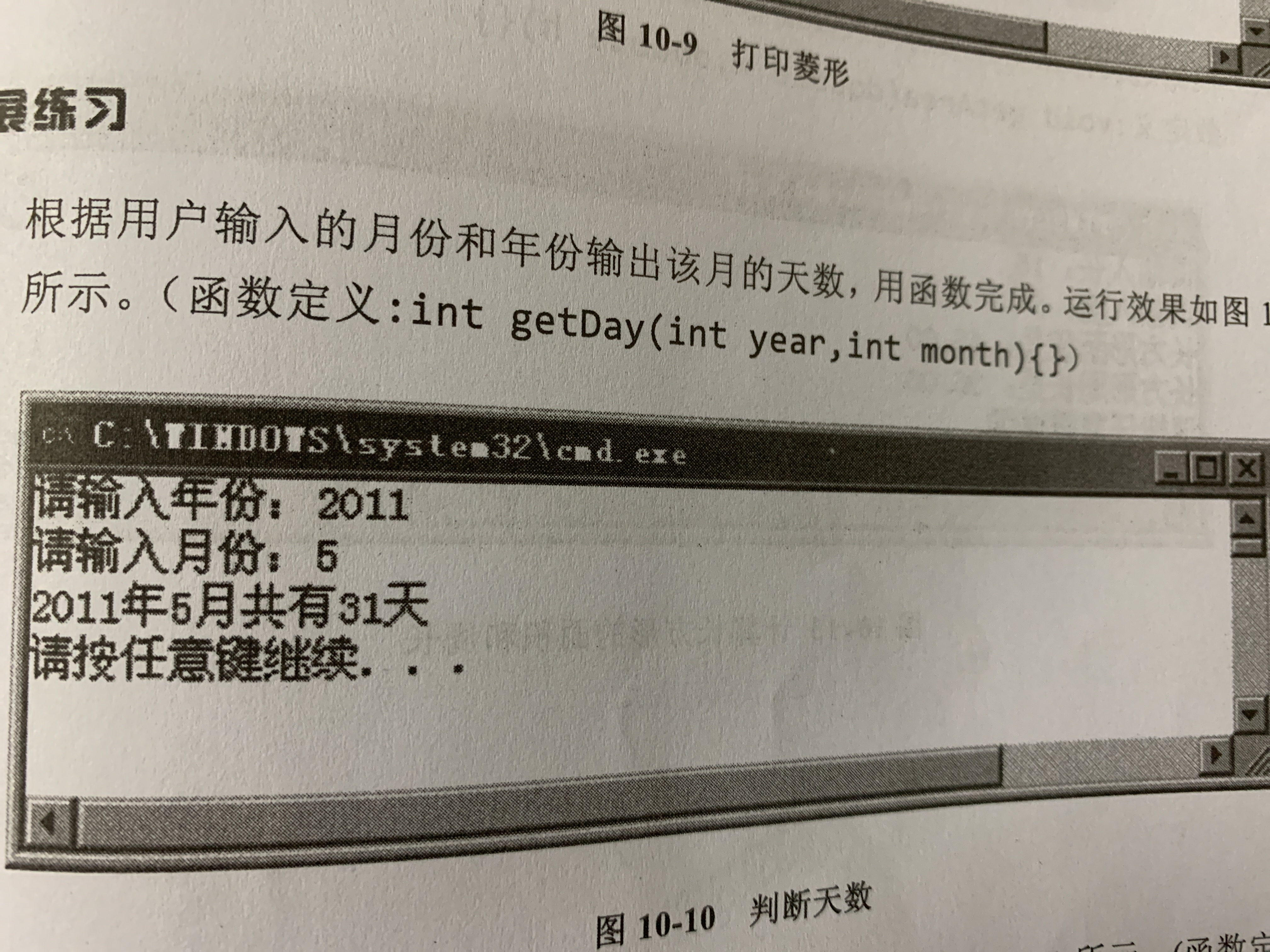2021-07-04 21:00

# 用函数完成如何写代码• 好问题 提建议
• 收藏

#### 1条回答默认 最新

•qfl_sdu 2021-07-04 21:08
已采纳

代码如下：

``````#include <stdio.h>
int days(int y,int m)
{
if (m == 1 || m == 3 ||m == 5 ||m == 7 ||m == 8 ||m == 10 ||m == 12)
{
return 31;
}else if (m == 4 ||m == 6 ||m == 9 ||m == 11)
{
return 30;
}else
{
if(y%4==0 && y%100!=0 || y%400==0)
return 29;
else
return 28;
}
}

int main()
{
int y,m;
printf("请输入年份:");
scanf("%d",&y);
printf("请输入月份:");
scanf("%d",&m);
printf("%d年%d月共有%d天\n",y,m,days(y,m));
return 0;
}
``````
已采纳该答案
评论
解决 无用
打赏 举报